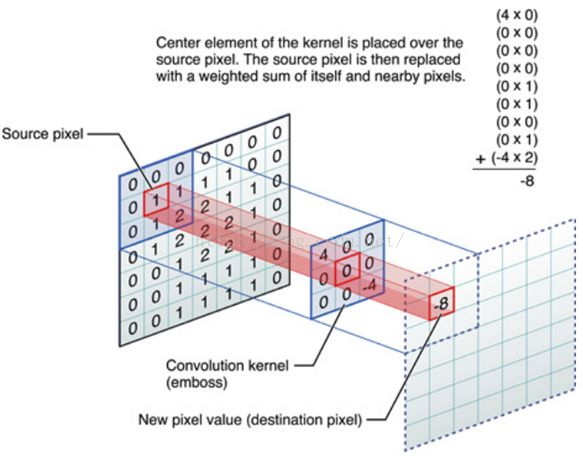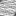# Deformable convolution layer with 'variable filter'

I think that deformable convolution is a relatively creative idea.
But what I feel is insufficient is that the size of the convolution kernel cannot be changed.
How to implement such a convolution layer in PyTorch:

1. Deformable convolution.

2. Variable convolution kernel size.

3. (If point 2 does not make sense in mathematical theory,)
Variable size for the image which put into the convolution kernel.
(When putting into the convolution kernel, the image will be resized to adapt to the convolution layer.)

I mean, how to implement such a convolution layer in PyTorch:

• point 1 and point 2

or

• point 1 and point 3

For the point 3, add a note.My question is whether the size of the convolution kernel can also be changed dynamically.
If it doesn’t make sense in mathematical theory, I think it’s useful to dynamically adjust the size of the cut image. Then I mean the cut image is before the image with source pixel as the picture shows. The cut image will be changed the size (the same as the size of the image with source pixel in the picture) and put into the convolution kernel.

Hi,

1. Could you explain exactly what a “Deformable Convolution” should compute? And what are the parameters?

2. You can do variable kernel size by doing a custom module that computes the new kernel during the forward pass and then calls the convolution operation with the new kernel size.

3. Here again you can do a custom module that will change the input size by interpolating the new values.

• What a “Deformable Convolution” should compute.

I want to deal with CT images of lung nodules and predict its benign and malignant like the image below. The point is that features in physiological medical images tend to vary widely. So I want to use “Deformable Convolution” to adapt to that.

It is difficult to determine the size of the image cut.
As shown below (size of 16 * 16, 32 * 32, 48 * 48), too big or too small is not appropriate.• What are the parameters.
• the size of image cut. (I think the variable size is the best.)
In fact, it is difficult to figure out the best segmentation size artificially.
• the size of kernel. (I think the variable size is the best.)
(The point is that features in physiological medical images tend to vary widely.)
• weights in the kernel. (There must be.)
• offset of the convolution kernel. (In fact, it’s the offset parameters of the “Deformable Convolution”)

I’m sorry, I do not understand. If the kernel size is variable, I mean, they will be independent.
Suppose there are three different sizes of convolution kernels: A = (3 * 3), B = (5 * 5), C = (7 * 7).
When the model thinks A is suitable, then it will use kernel A to train and update the weights in A. But it won’t update the weights in B and C. This is my understanding.

• Can such model work well?
• How can we code a model like this in PyTorch? (`if...else...` in `forward` function?)
• Or is my understanding wrong?

I’m so sorry, I don’t understand…

You can have a model with conditionals with no problem in pytorch:

``````class MyMod(nn.Module):
def __init__(self, params):
self.A = MyModA()
self.B = MyModB()
self.C = MyModC()

def forward(self, input):
cond = compute_condition(input)
if cond == 0:
return self.A(input)
elif cond == 1:
return self.B(input)
else:
return self.C(input)
``````

Now whether such model will work well for your task I don’t know. I’m afraid you will have to try and see experimentally if it does.

1 Like

Thanks for your suggestion.I will try it.@albanD & @shirui-japina
There is a great reference for understanding Deformable Convolution Operator: